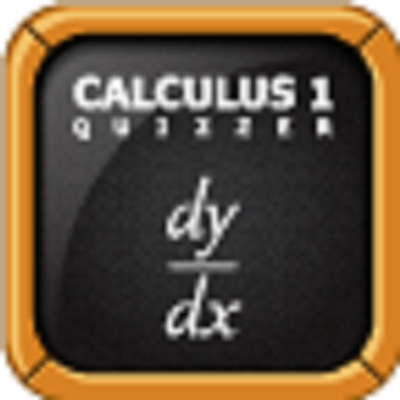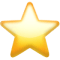Search app# Calculus 1 Quizzer

(1)
Price
\$2.99
Category
Education & Business
Last Update
None
View in store

#### Store Performance Index

64%

Store Performance Index shows overall performance of your app on app stores. It is based on combined ASO & reviews metrics.

See more data

## Ratings & Reviews performance for Calculus 1 Quizzer

Ratings & Reviews performance provides an overview of what users think of your app. Here are the key metrics to help you identify how your app is rated by users and how successful is your review management strategy.

Number of reviews,
total

1

Avg rating,
total5.0

#### Description

3980 chars

Calculus 1 Quizzer is a quiz application for students planning on or currently taking a college or university level Calculus 1 class. The application has 16 different levels. Each time you take a quiz, (even if you stay in the same level), the questions will be different. Each question has a tip to help the user solve the problem. It also has multiple profile selection and score tracking capabilities. The following are the topics covered in each level: 1. Functions: Introduction to when an equation is a function (horizontal line test). Includes trigonometric functions and polynomials as a pre-calculus review. Also covers linear functions, inverse functions, even-odd functions, rational functions, exponential functions, logarithms, etc.  Finally some basic concepts such as domain and range of a function and inverses. 2. Limits and Continuity Limits are usually the first thing covered in calculus, and would be best to be introduced early.  Convergence and divergence are central to this level. Includes limits of polynomials, rational functions, and trigonometric functions.  3. Differentiability and Derivative of a Function The definition of derivative  ( lim h->o of [f(x+h)-f(x)]/h ) depends on limits and it is best that students understand limits of functions first. This level covers the concept of a derivative as the slope of a function, basic properties of taking limits, tangent and normal lines, and how to calculate a derrivative using the lim h->0 method. 4. Power Rule, Product Rule and Quotient Rule This level simplifies the computation of derivatives using the above rules.  It also covers the derrivatives of powers, polynomials, and rational functions. 5. Derivatives by the Chain Rule This level covers the derivatives of composite functions. It also covers derivatives of logarithms and exponential functions. 6. Derivative of the trigonometric functions This level covers the derivatives of Sine, Cosine, tangent, cotangent, secant, and cosecant.  Also allows for practice of the previously learned rules. 7. Inverse Functions and their derivatives This level reviews when a function is invertible (horizontal line test). 8. Second Derivatives, concavity, and physical applications This level introduces the notion of a second derivative and covers the concept of concavity. 9. Graphs of functions and curve Sketching This level covers extreme value theorem, mean value theorem, relative max and mins by first and second derrivative tests, inflection points, vertical and horizontal asymptotes, increasing/decreasing intervals, concave up and concave down. 10. Implicit Differentiation This level covers the concepts of taking the derivative of an ellipse, hyperbola, circle, and other things that are not defined as functions but rather are defined by implicit equations (such as x^2 + y^2 = 1 for the circle). 11. Related Rates and Optimization This level focuses on the applications of implicit differentiation.  It also reviews simple geometric formulas such as area of a circle, sphere, triangle, cone, etc. 12. Linear Approximation and differentials. This should be introduced by the standard definition f(x+a) = f(a) + f'(a)(x-a). This level also covers mean value theorem and intermediate value theorem. 13. Area under a curve and Riemann sums This level covers the concept of left, middle, and right Riemann sums with a finite number of rectangles. 14. Anti-derivatives and integration This level covers the Fundamental Theorem of Calculus and the relation of definite integrals and area under a curve. Basic properties of integration are also covered. 15. Integration by Substitution Method This level covers how to do u-substitution and when it is necesary (sometimes refered to as the inverse-chain rule), as well as integration. 16. Solid of a revolution This level covers the concept of a solid of a revolution as well as how to compute it's volume through disc/washer method and cylindrical shell method.

Why to reply to reviews?

User reviews affect conversion to installs and app rating. Featured and helpful reviews are the first to be noticed by users and in case of no response can affect download rate. This is why it is highly recommended to reply to them.#### About Calculus 1 Quizzer

Developed by Adiel Gonzalez.

Calculus 1 Quizzer is ranking in   Education & Business

Last update was at Noneand the current version is 2.1.

To see all other keys and revenue click here 469275274

Calculus 1 Quizzer have a 1a user reviews.

Calculus 1 Quizzer was released in the App Store. It is developed by Adiel Gonzalez, who have also released the following apps.

Calculus 1 Quizzer has 1 user reviews.​

Calculus 1 Quizzer has an average rating of 5.0. ​The latest version of Calculus 1 Quizzer 2.1​ ​​was ​released on None​.

You can download Calculus 1 Quizzer ​here